• 单片机入门教程简单单片机入门教程简单单片机入门教程简单单片机入门教程简单单片机入门教程简单单片机入门教程简单单片机入门教程简单单片机入门教程简单单片机入门教程简单
• 这是面向新手的单片机教程，语言幽默风趣，采用二人对话叙述，极具可读性。
• 51单片机入门教程（2）——实现流水灯一、搭建流水灯电路二、流水灯程序2.1 延时程序2.2 延时函数2.3 按字节寻址2.4 逻辑移位2.5 条件判断 一、搭建流水灯电路 在Proteus中搭建流水灯电路如图 二、流水灯程序 我们...
51单片机入门教程（2）——实现流水灯一、搭建流水灯电路二、流水灯程序2.1 延时程序2.2 延时函数2.3 按字节寻址2.4 逻辑移位2.5 条件判断
一、搭建流水灯电路
在Proteus中搭建流水灯电路如图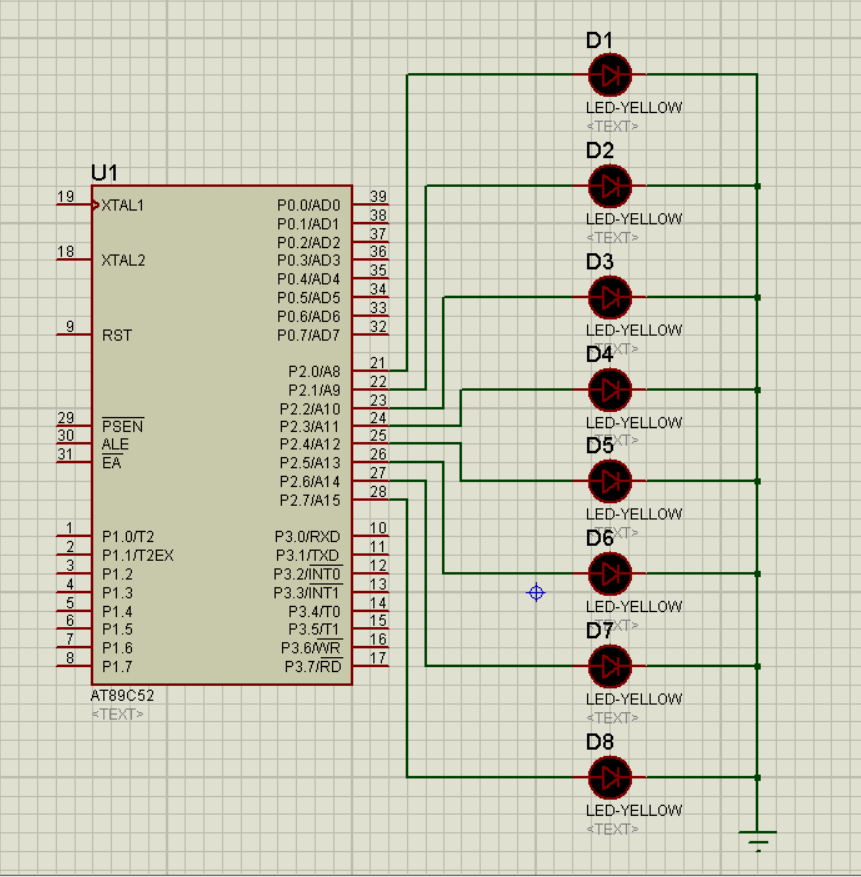二、流水灯程序
我们可以把流水灯看作依次点亮若干个灯。
程序如下：
#include <reg52.h>
sbit led1 = P2^0;
sbit led2 = P2^1;
sbit led3 = P2^2;
sbit led4 = P2^3;
sbit led5 = P2^4;
sbit led6 = P2^5;
sbit led7 = P2^6;
sbit led8 = P2^7;

void main()
{
//点亮第一个灯
led1 = 1;
led2 = 0;
led3 = 0;
led4 = 0;
led5 = 0;
led6 = 0;
led7 = 0;
led8 = 0;
//点亮第二个灯
led1 = 0;
led2 = 1;
led3 = 0;
led4 = 0;
led5 = 0;
led6 = 0;
led7 = 0;
led8 = 0;
//点亮剩余的灯
//省略……
while(1);
}

编译并下载程序到仿真中，观察现象发现只有第二个灯是亮的？？？
什么鬼？？？
2.1 延时程序
单片机的执行指令速度非常快，一个晶振是12MHz的单片机执行一条指令的速度是微秒级的，所以点亮第一个灯的时间太短了，以至于我们根本没有察觉。
因此我们需要一个延时的语句。
实现延时的方法就是循环执行很多次空指令。程序如下：
//延时一秒的程序
int i,j;
for(i = 0;i < 110; ++i)
{
for(j = 0; j < 1000; ++j)
{
;//什么也不做
}
}


然后我们就可以把流水灯的程序改成这样的：
#include <reg52.h>
sbit led1 = P2^0;
sbit led2 = P2^1;
sbit led3 = P2^2;
sbit led4 = P2^3;
sbit led5 = P2^4;
sbit led6 = P2^5;
sbit led7 = P2^6;
sbit led8 = P2^7;

void main()
{
int i,j;
//点亮第一个灯
led1 = 1;
led2 = 0;
led3 = 0;
led4 = 0;
led5 = 0;
led6 = 0;
led7 = 0;
led8 = 0;
//延时1秒
for(i = 0;i < 110; ++i)
{
for(j = 0; j < 1000; ++j)
{
;//什么也不做
}
}
//点亮第二个灯
led1 = 0;
led2 = 1;
led3 = 0;
led4 = 0;
led5 = 0;
led6 = 0;
led7 = 0;
led8 = 0;
//点亮剩余的灯
//省略……
while(1);
}

编译并下载程序到仿真中，观察现象发现首先第一个灯亮，过了一会儿第二个灯亮。
2.2 延时函数
我们剩下的任务就是依次点亮每个灯，但是每次点亮一个灯就需要写一段延时程序，很麻烦!
为了程序的可读性（toulan）,可以把延时程序写成一个子函数，随时供我们使用。
C语言中子函数的定义方式如下
返回值类型 函数名 (参数1，参数2，……)
{
函数体;
}

这样我们就可以把延时函数写成这样：
void delay1s()
{
int i,j;
for(i = 0; i<110;++i)
{
for(j = 0; j<1000;++j)
{
//什么也不做
}
}
}

几点说明：

void：因为该延时函数不需要返回值，所以写为void
delay1s：该函数的函数名，命名需要符合C语言的标识符命名规则。
(): 不需要传入参数，所以括号中为空
至此我们可以把流水灯程序写为以下形式：

#include <reg52.h>

sbit led1 = P2^0;
sbit led2 = P2^1;
sbit led3 = P2^2;
sbit led4 = P2^3;
sbit led5 = P2^4;
sbit led6 = P2^5;
sbit led7 = P2^6;
sbit led8 = P2^7;

//延时1s
void delay1s()
{
int i ,j;
for(i = 0;i<110; ++i){
for(j = 0;j<1000;++j){
;
}
}
}

void main()
{
//点亮第一个灯
led1 = 1;
led2 = 0;
led3 = 0;
led4 = 0;
led5 = 0;
led6 = 0;
led7 = 0;
led8 = 0;

//延时1s
delay1s();

//点亮第二个灯
led1 = 0;
led2 = 1;
led3 = 0;
led4 = 0;
led5 = 0;
led6 = 0;
led7 = 0;
led8 = 0;
//点亮剩余的灯
//省略……
while(1);
}

2.3 按字节寻址
我们可以看到，上面的代码十分冗长，每次点亮一个灯需要8条语句，那么如何简化？
比如把
led1 = 1;led2 = 0; led3 = 0; led4 = 0; led5 = 0; led6 = 0; led7 = 0; led8 = 0;
这8条语句替代为P2 = 0000 0001？？？
答案是可以的。代码如下
unsigned char a = 0x01;  //0x01是0000 0001的16进制形式
P2 = a;//相当于led1 = 1;led2 = 0; led3 = 0;  led4 = 0; led5 = 0; led6 = 0; led7 = 0; led8 = 0;

至此，我们可以把流水的代码优化为如下形式：
#include <reg52.h>

//延时1s
void delay1s()
{
int i ,j;
for(i = 0;i<110; ++i){
for(j = 0;j<1000;++j){
;
}
}
}

void main()
{
unsigned char a1 = 0x01 ;    // 0000 0001
unsigned char a2 = 0x02;    // 0000 0010
//点亮第一个灯
P2 = a1;

//延时1s
delay1s();

//点亮第二个灯
P2 = a2;
//点亮剩余的灯
//省略……
while(1);
}

2.4 逻辑移位
依次点亮8个灯，每点亮一个灯都需要一句赋值语句还是很麻烦 。
所以可以使用逻辑移位语句，每次赋值后，将数值左移一位。
C语言逻辑左移代码如下：
unsigned char a = 0x01;  //a = 0000 0001
unsigned char b = a<<1;  // b = 0000 0010
usingned char c = a<<3;   //c = 0000 1000

至此，我们可以把流水灯的代码优化如下：
#include <reg52.h>
//延时1s
void delay1s()
{
int i ,j;
for(i = 0;i<110; ++i){
for(j = 0;j<1000;++j){
;
}
}
}

void main()
{
//初始化
unsigned char a = 0x01;
while(1)
{
//循环点亮流水灯
P2 = a;
a = a<<1;
delay1s();
}
}

编译并下载程序到仿真中，观察现象发现8个灯依次亮过之后不再亮了。
2.5 条件判断
因为在移位操作中，当变量a的值为1000 0000时，再次执行左移操作，a 中的1就溢出了，因此a的值变为0000 0000，此时我们需要加一个判断，使a再次恢复为0000 0001
C语言中，if条件判断使用方式如下
if(判断条件)
{
//语句
}

当判断条件为真时，执行{ }中的语句。
至此，流水灯代码可改成如下形式：
#include <reg52.h>

//延时1s
void delay1s()
{
int i ,j;
for(i = 0;i<110; ++i){
for(j = 0;j<1000;++j){
;
}
}
}

void main()
{
unsigned char a = 0x01;
while(1)
{
if(a == 0x00)   //如果高位溢出
{
a = 0x01;      //则恢复
}
//循环点亮led灯
P2 = a;
a = a<<1;
delay1s();
}
}



展开全文嵌入式
• 瑞萨单片机入门教程 ，IDE及单片机的使用，实用好资源
• C51单片机入门教程，介绍了51单片机的基本眼里及C语言开发流程
• 51单片机入门教程,51单片机入门教程51单片机入门教程,51单片机入门教程
• 单片机 51测试网单片机入门教程教程51测试网单片机入门教程教程
• 51单片机入门教程(易懂版)pdf,51单片机入门教程(易懂版)
• STM8单片机入门教程_V2.3rar,STM8单片机入门教程_V2.3
• 义隆单片机入门教程义隆单片机入门教程义隆单片机入门教程
• 51单片机入门教程（1）——点亮一个LED灯 先放这里，下午再写
51单片机入门教程（1）——点亮一个LED灯
一、什么是单片机
单片机（Microcontrollers）是一种集成电路芯片，是采用超大规模集成电路技术把具有数据处理能力的中央处理器CPU、随机存储器RAM、只读存储器ROM、多种I/O口和中断系统、定时器/计数器等功能（可能还包括显示驱动电路、脉宽调制电路、模拟多路转换器、A/D转换器等电路）集成到一块硅片上构成的一个小而完善的微型计算机系统，在工业控制领域广泛应用。从上世纪80年代，由当时的4位、8位单片机，发展到现在的300M的高速单片机。
以上是百度百科上的词条，随便看看就行了，下面说一下我对单片机的理解：
我们都知道，我们的电脑是由CPU、内存、磁盘、IO设备等组成的，而单片机简而言之，就是一个微型电脑，它在一块芯片上包含了CPU，硬盘(flash)和内存条(ram)以及基本输入输出设备(io口)。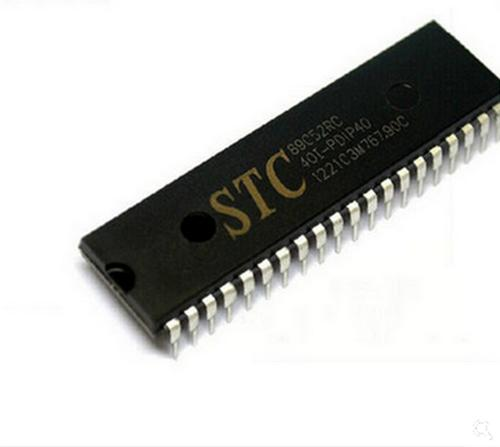二、怎么学习单片机
void 学习单片机()
{
while(1){
动手写单片机程序;
调试程序;
发现程序中的语法与逻辑错误;
改正程序中的错误;
}
}

三、点亮一个LED灯
说了这么多，现在步入正题。
3.1 在Proteus上搭建LED灯的电路
首先打开Proteus软件，界面如图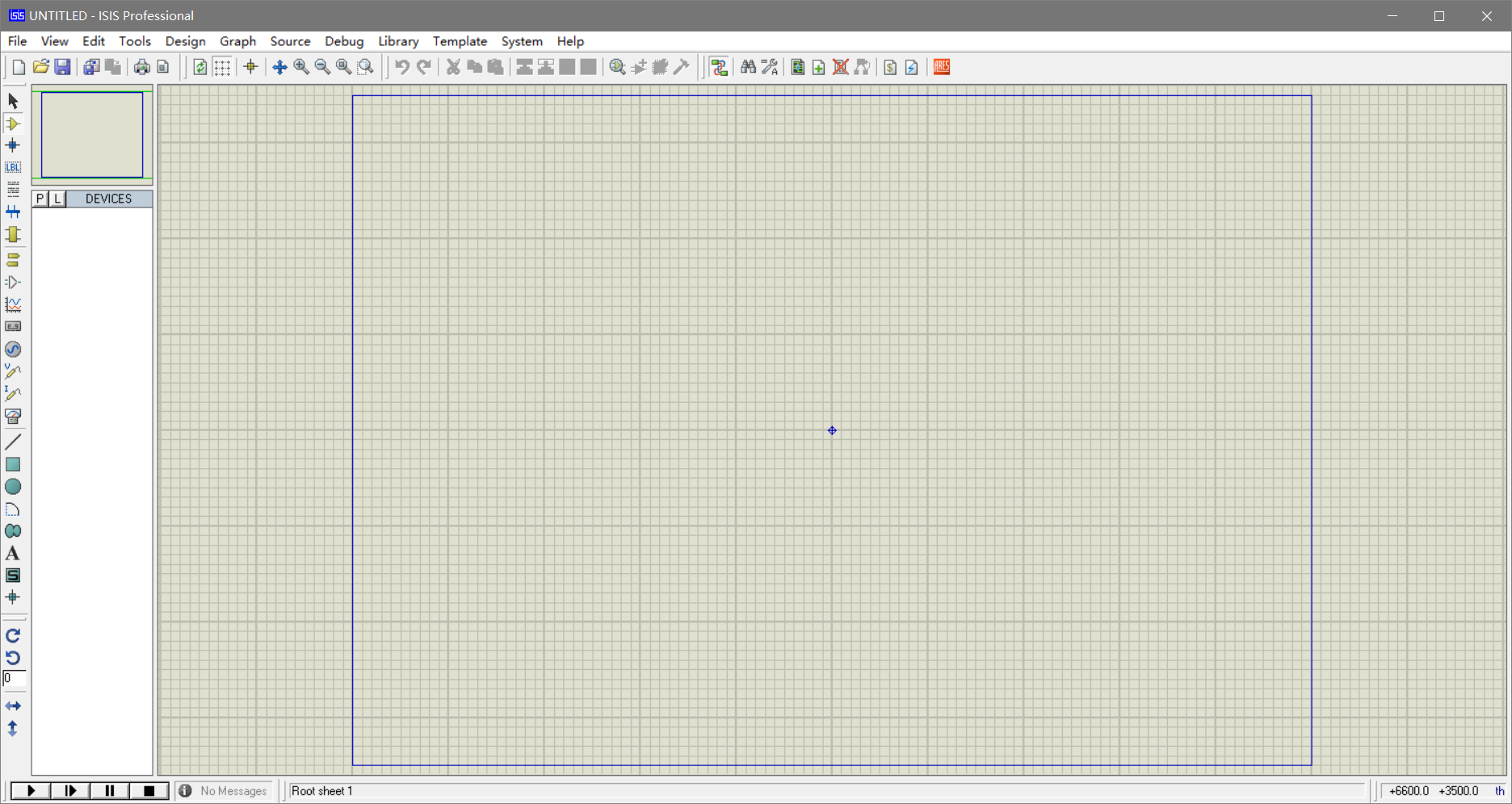左侧的一栏是各种类型的组建，其中我们主要需要用到的有组件选项（component mode）和终端选项（Terminals mode）。
组件选项包括了我们用到的芯片，元件和其它的一些东西。
终端选项包括了VCC、GND等一些组件。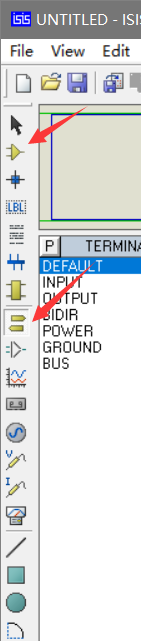首先在组件选项中点击“P”按钮，找到我们需要的89c51芯片，并放入到主面板里。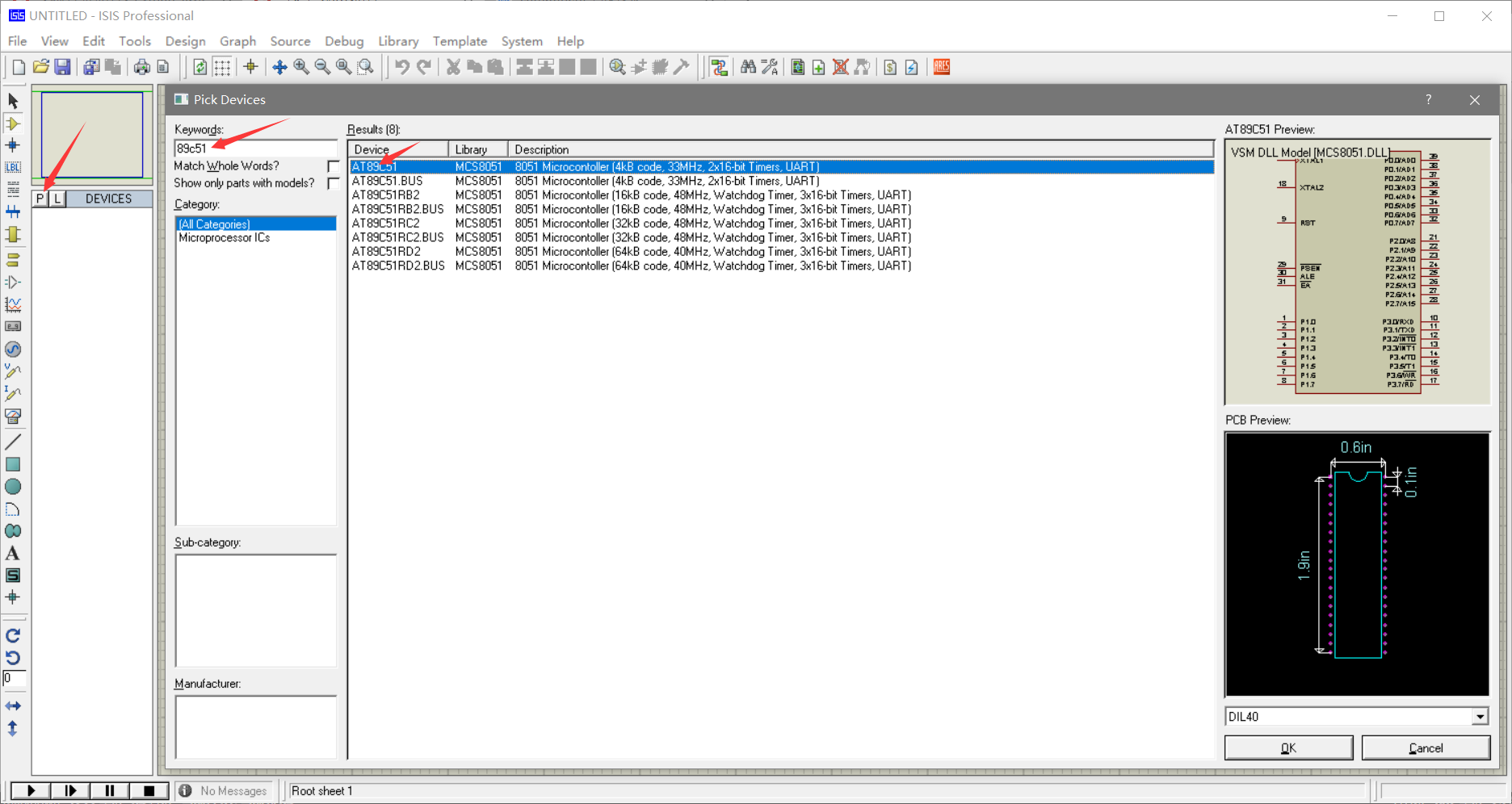同理
在组件选项中输入LED-YELLOW找到led灯放入主面板
在终端选项中选择POWER放入到主面板。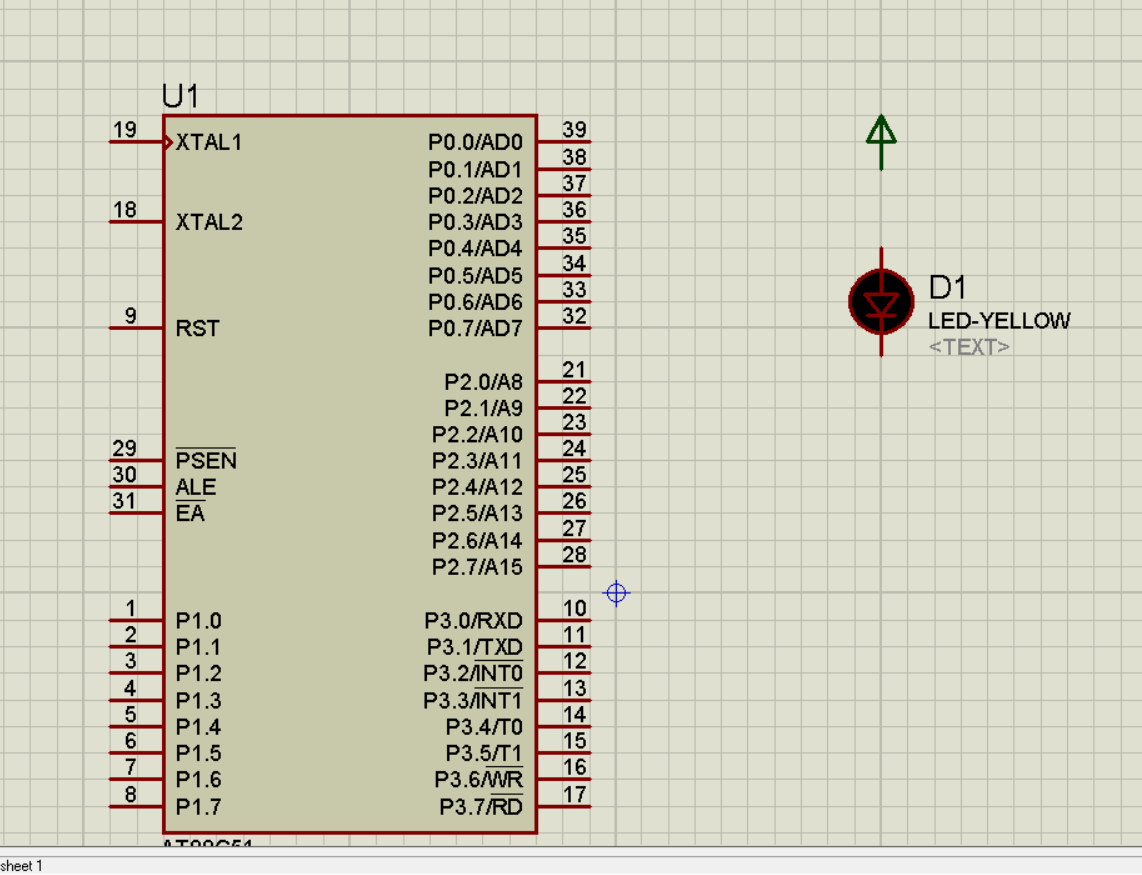然后就要连接电路。power为电路提供电源输入，即电路的正极，所以将power的线连接到led灯的正极，然后我们把led灯的另一端接到单片机的P2^0引脚上。
之后我们就可以在程序中给P2^0引脚一个低电平（即电路的负极）使其构成一个回路，就可以达到点亮led灯的目的。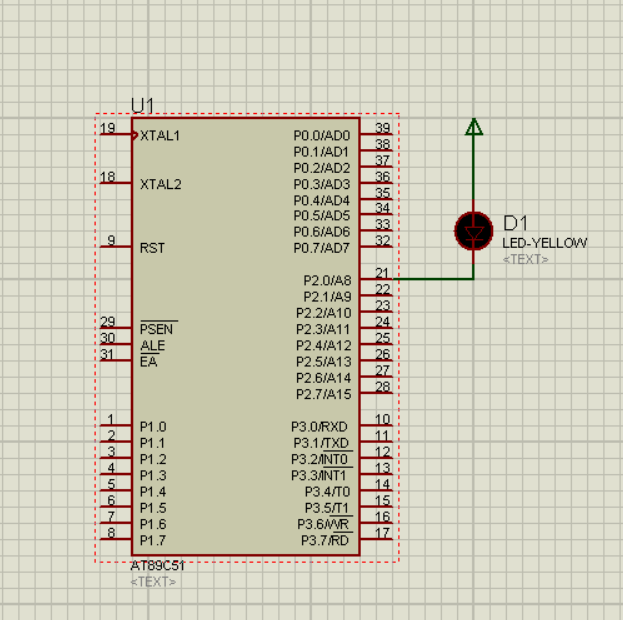3.2 在keil中创建工程
3.2.1新建一个工程
打开keil，在菜单栏中找到工程（project），点击第一个，然后选择工程保存路径并点击保存。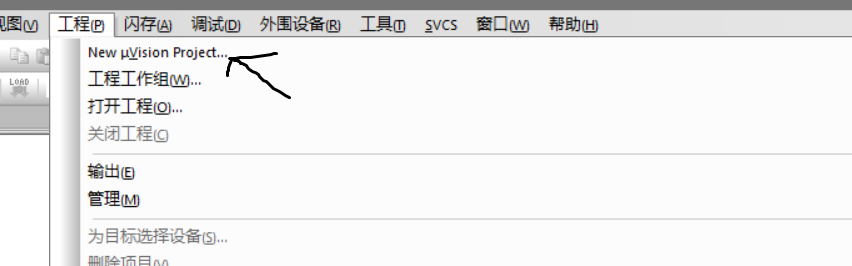然后选择单片机型号，找到Atmel点击左边的小加号，选择at89c51（或at89c52），点击确定。
j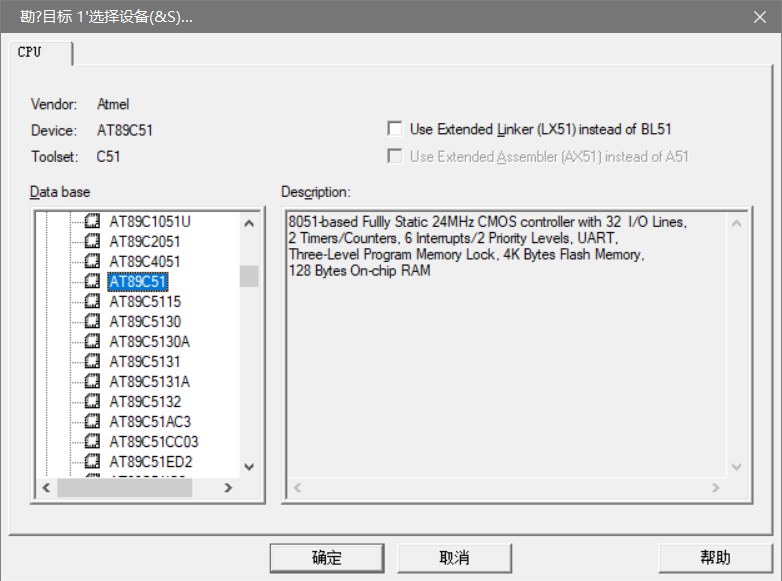接下来的窗口是提示我们“是否把启动代码添加到工程中”，我们选择确定。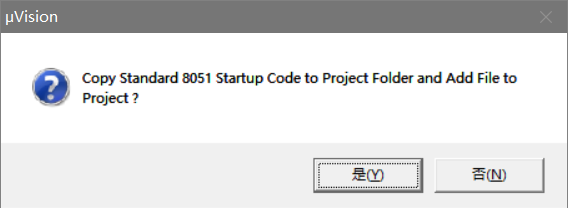这样我们的工程就创建好了。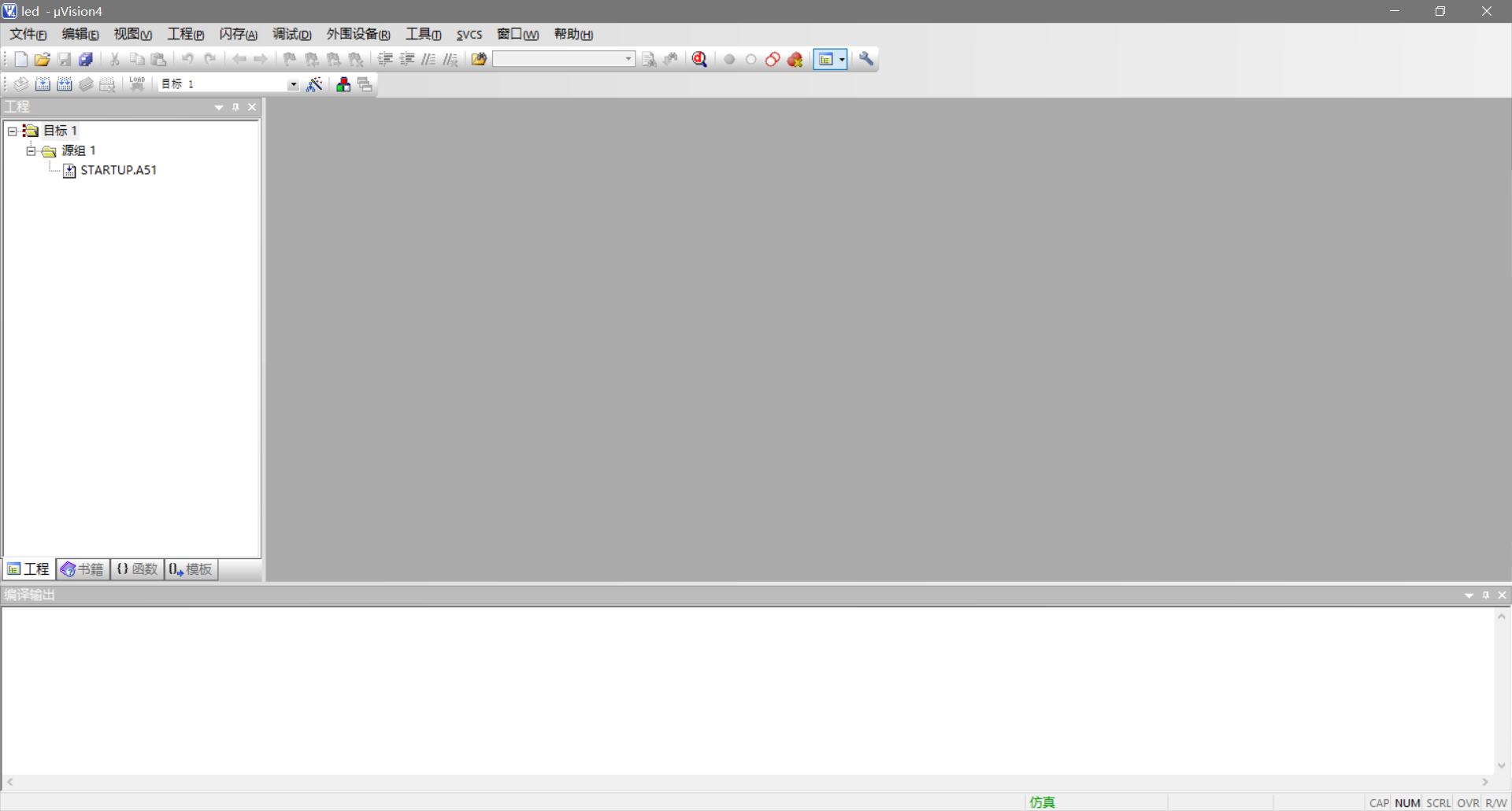随后我们需要给工程创建源码文件。
点击“文件”->“新建”，使用组合键ctrl+s保存文件，命名为main.c（可以随便命名，但是后面要加上 .c），点击保存。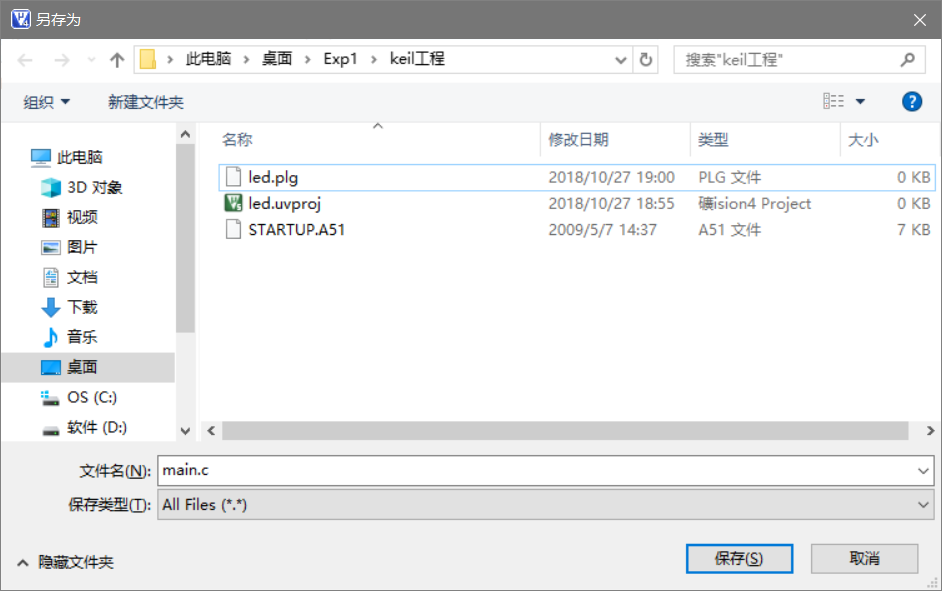右击源组，点击添加文件到源组。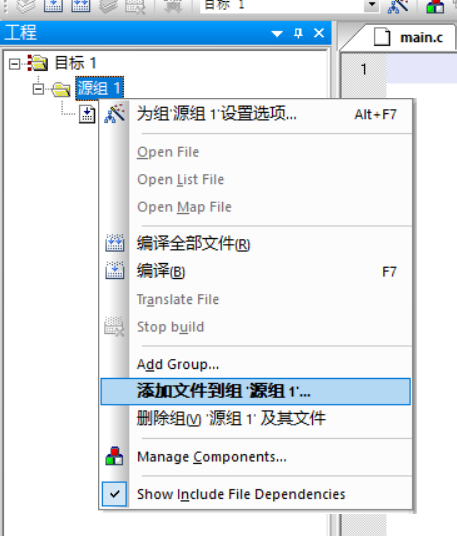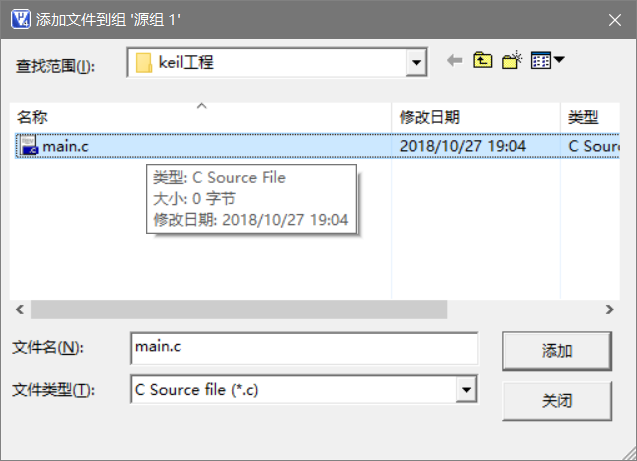点击这里的魔术棒，选择output，选中create hex file。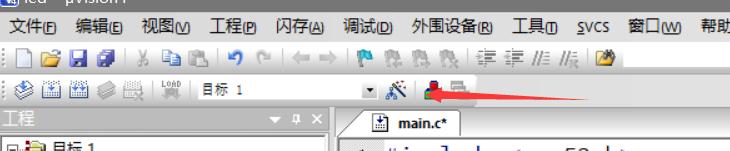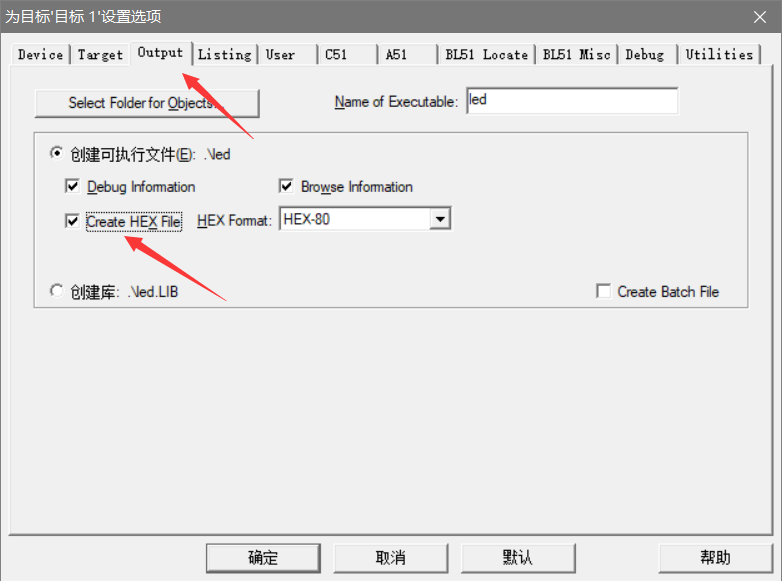至此就可以编写代码了。
在文件中输入以下代码:
#include <reg52.h>
sbit led = P2^0;
void main(){
while(1){
led = 0;
}
}

说一下这几句代码是什么意思。

第一行是包含头文件，头文件里面定义了单片机寄存器的一些定义。初学者不理解也没有关系。
第二行是声明了P2^0端口，相当于给这个端口起了一个名字。
第三行是main函数，代码从这里开始执行。
第四行是一个while死循环，程序会一直执行while循环里面的内容。
第五行是给名字为led的端口（即P2^0端口）一个低电平（1代表高电平，0代表低电平），使led灯的两端构成回路。

然后编译程序，点击左上角的编译按钮。可以看到"0 error , 0 warning"的提示。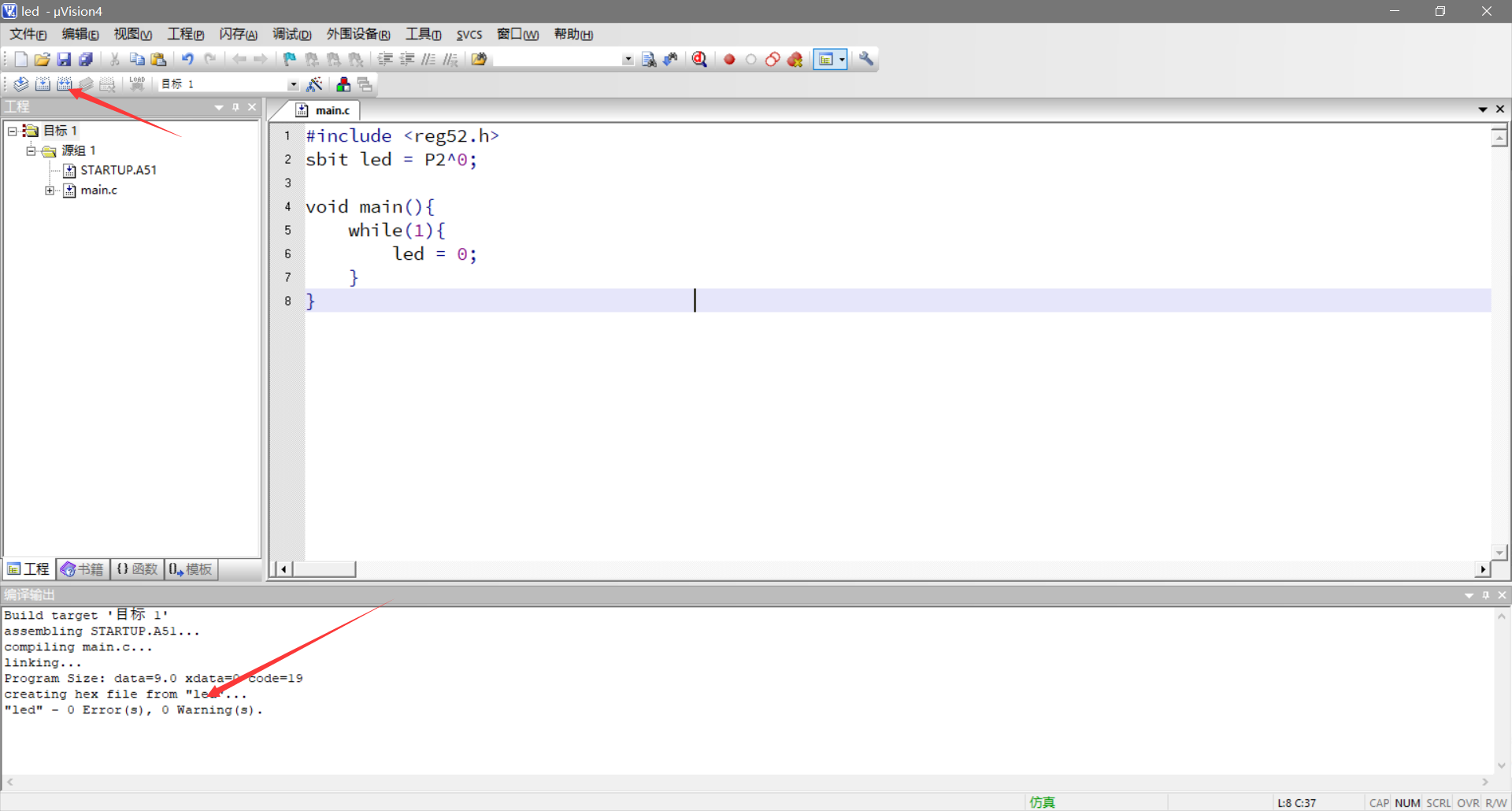3.3 将程序导入到单片机中运行
我们已经搭建好了电路，写好了程序，生成了hex文件，之后需要在电路上运行我们的程序。
打开3.1中创建的仿真电路，双击单片机，找到3.2生成的hex文件，点击打开。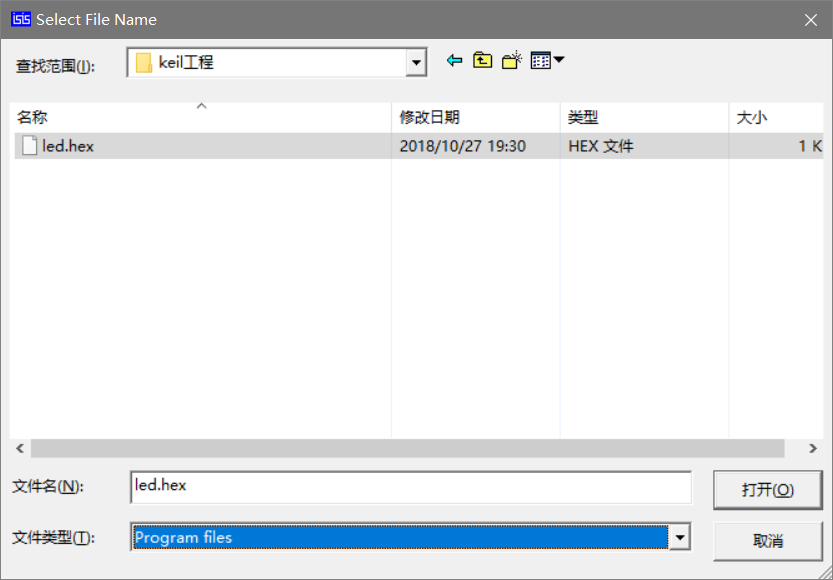左下角的一排控制按钮意思分别是运行 单步 暂停 结束，我们点击运行就可以看到led灯被点亮。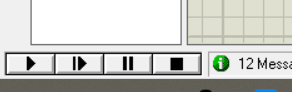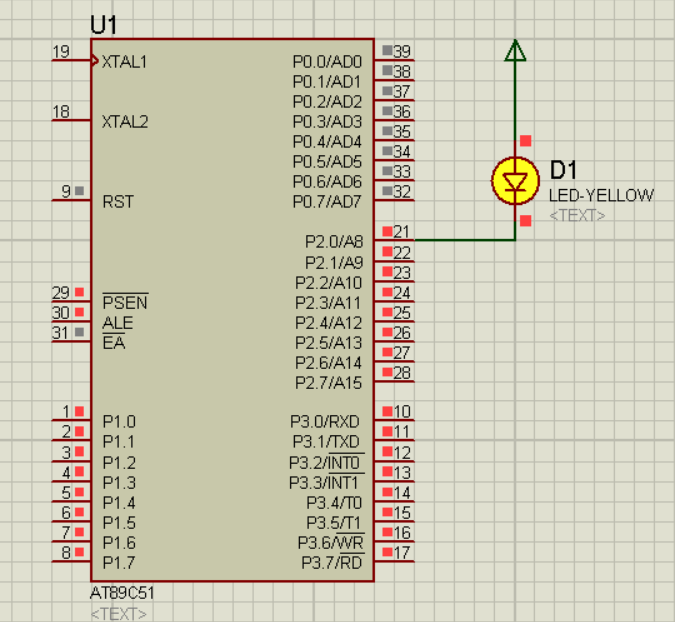展开全文嵌入式...